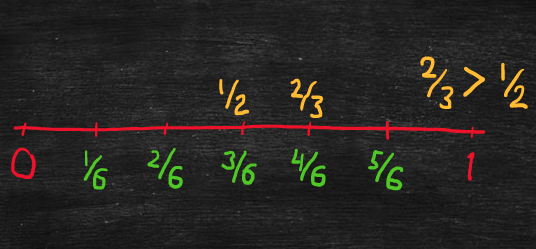Part 1: Numbers

# 1.4: Comparing fractions using a number line

Notes

In the following, “A/B” represents the fraction “A over B.” For example, “2/3” refers to the fraction “2 over 3” or “two-thirds.”

A number line is a useful tool for comparing fractions to determine which of two fractions is greater. For example,

• To compare 1/2 and 2/3, we need to express the fractions using a common denominator.
• A common denominator for 2 and 3 is 6, and 1/2 = 3/6 and 2/3 = 4/6.
• Draw a number line between 0 and 1, divide the line into sixths, and mark 1/2 = 3/6 on the third tick mark and 2/3 = 4/6 on the fourth tick mark.
• Thus, 2/3 > 1/2.For another example,

• To compare 5/8 and 3/4, a common denominator for 8 and 4 is 8, and 5/8 does not change, while 3/4 = 6/8.
• Draw a number line between 0 and 1, divide the line into eighths, and mark 5/8 on the fifth tick mark and 3/4 = 6/8 on the sixth tick mark.
• Thus, 3/4 > 5/8.

For another example,

• To compare 5/6 and 3/4, a common denominator for 6 and 4 is 12, and 5/6 = 10/12, while 3/4 = 9/12.
• Draw a number line between 0 and 1, divide the line into twelfths, and mark 5/6 = 10/12 on the tenth tick mark and 3/4 = 9/12 on the ninth tick mark.
• Thus, 5/6 > 3/4.

The video below shows how to use number lines to compare fractions.

Practice Exercises

Do the following exercises to practice ordering fractions. Visualize the fractions on a number line to help you determine where to place the fractions in order from least on the left to greatest on the right.